Updating search results...

# 1528 Results

View
Selected filters:
• EngageNY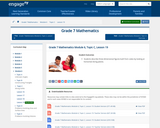Conditional Remix & Share Permitted
CC BY-NC-SA
Rating
0.0 stars

In this lesson, students describe three-dimensional figures built from cubes by looking at horizontal slicing planes.

Subject:
Mathematics
Material Type:
Lesson Plan
Provider:
EngageNY
02/09/2017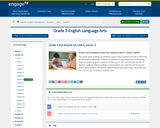Conditional Remix & Share Permitted
CC BY-NC-SA
Rating
0.0 stars

In this lesson, students continue to build their reading skills by asking questions and reading sections of the text, Everything You Need to Know about Frogs and Other Slippery Creatures. Students continue to build their general knowledge about frogs by reading selections about life cycle, skin, and habitat.

Subject:
English Language Arts
Material Type:
Lesson Plan
Provider:
EngageNY
04/19/2017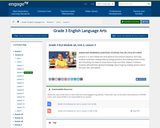Conditional Remix & Share Permitted
CC BY-NC-SA
Rating
0.0 stars

In this lesson, students build their reading skills by asking questions and reading sections of the text, Everything You Need to Know about Frogs and Other Slippery Creatures. Students build their general knowledge about frogs by reading selections about life cycle, skin, and habitat.

Subject:
English Language Arts
Material Type:
Lesson Plan
Provider:
EngageNY
04/19/2017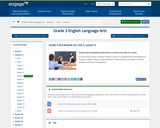Conditional Remix & Share Permitted
CC BY-NC-SA
Rating
0.0 stars

In this lesson, students continue to build their reading skills by asking questions and reading sections of the text, Everything You Need to Know about Frogs and Other Slippery Creatures. Students continue to build their general knowledge about frogs by reading selections about life cycle, skin, and habitat.

Subject:
English Language Arts
Material Type:
Lesson Plan
Provider:
EngageNY
04/19/2017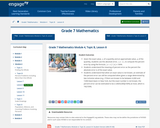Conditional Remix & Share Permitted
CC BY-NC-SA
Rating
0.0 stars

Given the exact value, x, of a quantity and an approximate value, a, of the quantity, students use the absolute error, | a - x |, to compute the percent error by using the formula | a - x |/ |x| x 100%.
Students understand the meaning of percent error as the percent the absolute error is of the exact value.
Students understand that when an exact value is not known, an estimate of the percent error can still be computed when given a range determined by two inclusive values (e.g., if there are known to be between 6,000 and 7,000 black bears in New York, but the exact number is not known, the percent error can be estimated to be (1,000/6,000)(100%) at most, which is 16(2/3)%).

Subject:
Mathematics
Material Type:
Lesson Plan
Provider:
EngageNY
01/30/2017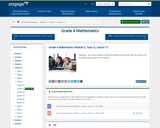Conditional Remix & Share Permitted
CC BY-NC-SA
Rating
0.0 stars

For this lesson, students use visual models to add and subtract two fractions with the same units including subtracting from one whole.

Subject:
Mathematics
Material Type:
Lesson Plan
Provider:
EngageNY
02/24/2017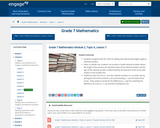Conditional Remix & Share Permitted
CC BY-NC-SA
Rating
0.0 stars

In this lesson, students recognize that the rules for adding and subtracting integers apply to rational numbers. Given a number line, students use arrows to model rational numbers where the length of the arrow is the absolute value of the rational number and the sign of the rational number is determined by the direction of the arrow with respect to the number line. Students locate the sum p + q of two rational numbers on a number line by placing the tail of the arrow for q at p and locating p + q at the head of the arrow. They create an arrow for the difference p - q by first rewriting the difference as a sum, p + (- q), and then locating the sum.

Subject:
Mathematics
Material Type:
Lesson Plan
Provider:
EngageNY
01/24/2017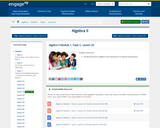Rating
0.0 stars

Students perform addition and subtraction of rational expressions.

Subject:
Mathematics
Material Type:
Lesson Plan
Provider:
EngageNY
02/02/2018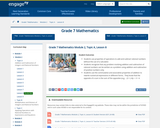Conditional Remix & Share Permitted
CC BY-NC-SA
Rating
0.0 stars

In this lesson, students use properties of operations to add and subtract rational numbers without the use of a calculator. Students recognize that any problem involving addition and subtraction of rational numbers can be written as a problem using addition and subtraction of positive numbers only. Students use the commutative and associative properties of addition to rewrite numerical expressions in different forms. They know that the opposite of a sum is the sum of the opposites (e.g., - (3 + (-4)) = -3 + 4.

Subject:
Mathematics
Material Type:
Lesson Plan
Provider:
EngageNY
01/24/2017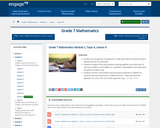Conditional Remix & Share Permitted
CC BY-NC-SA
Rating
0.0 stars

In this lesson, students use properties of operations to add and subtract rational numbers without the use of a calculator. Students recognize that any problem involving addition and subtraction of rational numbers can be written as a problem using addition and subtraction of positive numbers only. Students use the commutative and associative properties of addition to rewrite numerical expressions in different forms. They know that the opposite of a sum is the sum of the opposites; e.g., - ( 3 - 4) = -3 + 4.

Subject:
Mathematics
Material Type:
Lesson Plan
Provider:
EngageNY
01/24/2017Conditional Remix & Share Permitted
CC BY-NC-SA
Rating
0.0 stars

In this lesson, students understand addition of integers as putting together or counting up, where counting up a negative number of times is counting down. Students use arrows to show the sum of two integers, p + q, on a number line and to show that the sum is distance | q | from p to the right if q is positive and to the left if q is negative. Students refer back to the Integer Game to reinforce their understanding of addition.

Subject:
Mathematics
Material Type:
Lesson Plan
Provider:
EngageNY
01/24/2017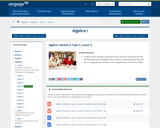Rating
0.0 stars

Lesson showing how to factor quadratics specifically when leading coefficient is not 1.

Subject:
Mathematics
Material Type:
Lesson Plan
Provider:
EngageNY
04/02/2018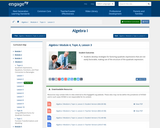Rating
0.0 stars

Lesson with application of factoring quadratic expressions and word problems.

Subject:
Mathematics
Material Type:
Lesson Plan
Provider:
EngageNY
04/02/2018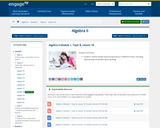Rating
0.0 stars

In this lesson, students rewrite simple rational expressions in different forms, including representing remainders when dividing.

Subject:
Mathematics
Material Type:
Lesson Plan
Provider:
EngageNY
07/27/2018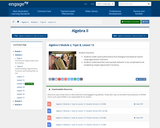Rating
0.0 stars

In this lesson, students model cyclical phenomena from biological and physical science using trigonometric functions. Students understand that some periodic behavior is too complicated to be modeled by simple trigonometric functions.

Subject:
Mathematics
Material Type:
Lesson Plan
Provider:
EngageNY
08/15/2018Rating
0.0 stars

In this lesson, students summarize data on two categorical variables collected from a sample using a two-way frequency table. Given a two-way frequency table, students construct a relative frequency table and interpret relative frequencies.

Subject:
Mathematics
Material Type:
Lesson Plan
Provider:
EngageNY
08/15/2018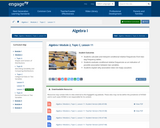Rating
0.0 stars

In this lesson, students summarize data on two categorical variables collected from a sample using a two-way frequency table. Given a two-way frequency table, students construct a relative frequency table and interpret relative frequencies.

Subject:
Mathematics
Material Type:
Lesson Plan
Provider:
EngageNY
08/15/2018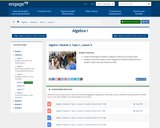Rating
0.0 stars

In this lesson, students distinguish between categorical data and numerical data. Students summarize data on two categorical variables collected from a sample using a two-way frequency table.

Subject:
Mathematics
Material Type:
Lesson Plan
Provider:
EngageNY
08/15/2018Rating
0.0 stars

In this lesson, students distinguish between scatter plots that display a relationship that can be reasonably modeled by a linear equation and those that should be modeled by a nonlinear equation.

Subject:
Mathematics
Material Type:
Lesson Plan
Provider:
EngageNY
08/15/2018